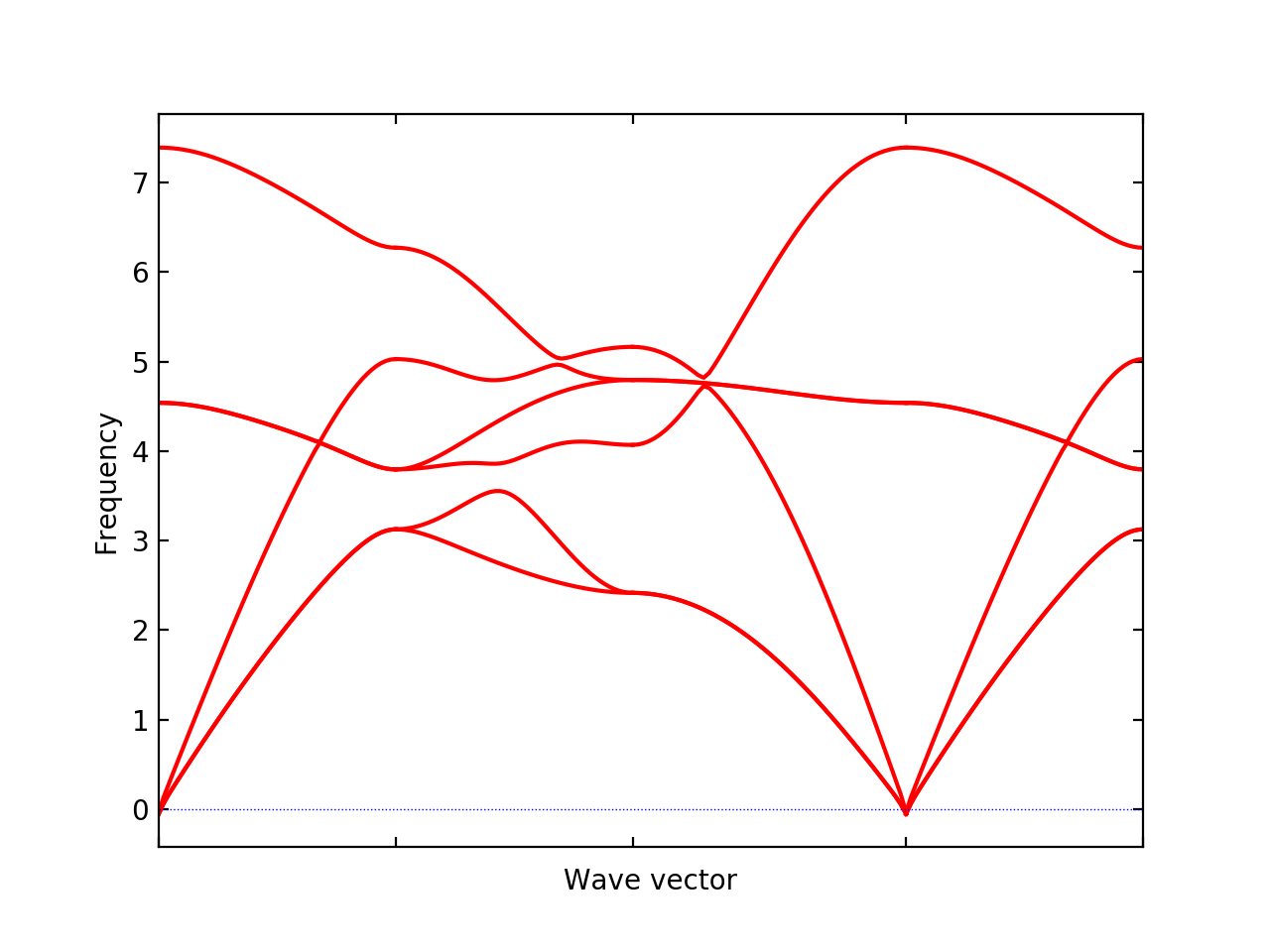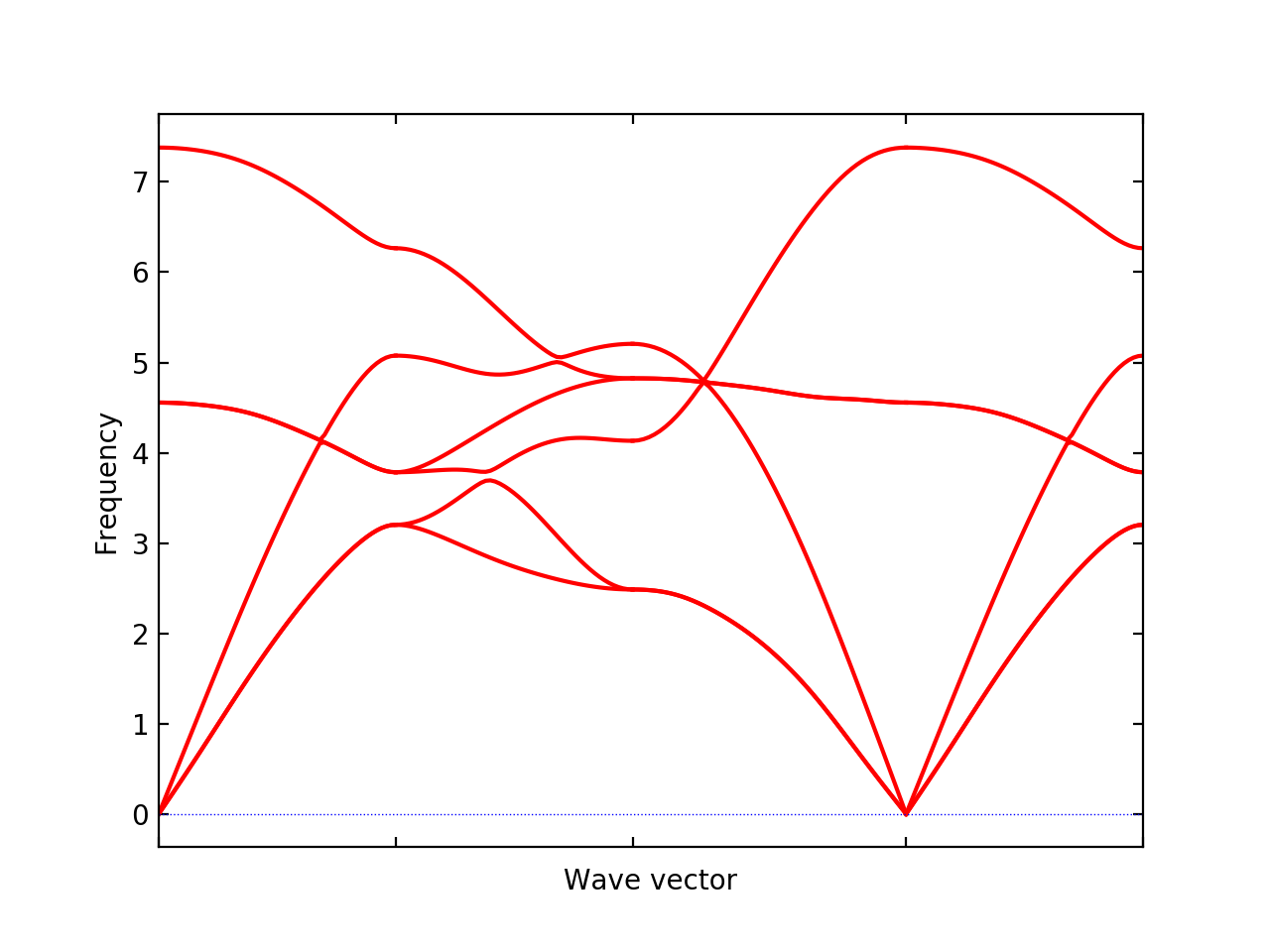# Quantum ESPRESSO (QE) & phonopy calculation#

Quantum ESPRESSO package itself has a set of the phonon calculation system. But the document here explains how to calculate phonons using phonopy, i.e., using the finite displacement and supercell approach.

## Supported QE-PW tags#

Currently QE-PW tags that phonopy can read are shown below. Only `ibrav = 0` type representation of crystal structure is supported. More tags may be supported on request.

```nat, ntyp, celldm(1), ATOMIC_SPECIES, ATOMIC_POSITIONS, CELL_PARAMETERS
```

## How to run#

The procedure of QE-phonopy calculation is shown below using the NaCl example found in `example/NaCl-QE` directory.

1. Read a QE-PW input file and create supercells with --qe option:

```% phonopy --qe -d --dim="2 2 2" -c NaCl.in
```

In this example, 2x2x2 supercells are created. `supercell.in` and `supercell-xxx.in` (`xxx` are numbers) give the perfect supercell and supercells with displacements, respectively. In the case of the NaCl example, two files `supercell-001.in` and `supercell-002.in` are created. In these supercell files, lines only relevant to crystal structures are given. `phonopy_disp.yaml` is also generated, which contains information about supercell and displacements.

2. To make QE-PW input files, necessary setting information is added to `supercell-xxx.in` files, e.g., by:

```% for i in {001,002};do cat header.in supercell-\$i.in >| NaCl-\$i.in; done
```

where `header.in` is specially made for this NaCl example and this file is found in `example/NaCl-QE` directory. This setting is of course dependent on systems and has to be written for each interested system. Note that supercells with displacements must not be relaxed in the force calculations, because atomic forces induced by a small atomic displacement are what we need for phonon calculation.

Then QE-PW supercell calculations are executed to obtain force on atoms, e.g., as follows:

```% mpirun pw.x -i NaCl-001.in |& tee NaCl-001.out
% mpirun pw.x -i NaCl-002.in |& tee NaCl-002.out
```
3. To create `FORCE_SETS`, that is used by phonopy, the following phonopy command is executed:

```% phonopy -f NaCl-001.out NaCl-002.out
```

Here `.out` files are the saved text files of standard outputs of the QE-PW calculations. If more supercells with displacements were created in the step 1, all `.out` files are given in the above command. To run this command, `phonopy_disp.yaml` has to be located in the current directory because the information on atomic displacements stored in `phonopy_disp.yaml` are used to generate `FORCE_SETS`. See some more detail at Quantum ESPRESSO interface.

4. Now post-process of phonopy is ready to run. The unit cell file used in the step 1 has to be specified but `FORCE_SETS` is automatically read. Examples of post-process are shown below.

```% phonopy --qe -c NaCl.in -p band.conf
_
_ __ | |__   ___  _ __   ___   _ __  _   _
| '_ \| '_ \ / _ \| '_ \ / _ \ | '_ \| | | |
| |_) | | | | (_) | | | | (_) || |_) | |_| |
| .__/|_| |_|\___/|_| |_|\___(_) .__/ \__, |
|_|                            |_|    |___/
1.13.0

Python version 2.7.14
Spglib version 1.10.3
Calculator interface: qe
Band structure mode
Settings:
Supercell: [2 2 2]
Primitive axis:
[ 0.   0.5  0.5]
[ 0.5  0.   0.5]
[ 0.5  0.5  0. ]
Spacegroup: Fm-3m (225)
Computing force constants...
max drift of force constants: -0.001194 (zz) -0.000000 (zz)
Reciprocal space paths in reduced coordinates:
[ 0.00  0.00  0.00] --> [ 0.50  0.00  0.00]
[ 0.50  0.00  0.00] --> [ 0.50  0.50  0.00]
[ 0.50  0.50  0.00] --> [-0.00 -0.00  0.00]
[ 0.00  0.00  0.00] --> [ 0.50  0.50  0.50]
...
````--qe -c NaCl.in` is specific for the QE-phonopy calculation but the other settings are totally common among calculator interfaces such as

```% phonopy --qe -c NaCl.in --dim="2 2 2" [other-OPTIONS] [setting-file]
```

For settings and command options, see Setting tags and Command options, respectively, and for examples, see Examples.

### Non-analytical term correction (Optional)#

To activate non-analytical term correction, BORN (optional) is required. This file contains the information of Born effective charge and dielectric constant. These physical values are also obtained from the PW (`pw.x`) & PH (`ph.x`) codes in Quantum ESPRESSO package. There are two steps. The first step is usual self-consistent field (SCF) calculation by and the second step is running its response function calculations under DFPT.

For the SCF calculation, the input file `NaCl.in` looks like:

``` &control
calculation = 'scf'
tprnfor = .true.
tstress = .true.
pseudo_dir = '/home/togo/espresso/pseudo/'
/
&system
ibrav = 0
nat = 8
ntyp = 2
ecutwfc = 70.0
/
&electrons
diagonalization = 'david'
conv_thr = 1.0d-9
/
ATOMIC_SPECIES
Na  22.98976928 Na.pbe-spn-kjpaw_psl.0.2.UPF
Cl  35.453      Cl.pbe-n-kjpaw_psl.0.1.UPF
ATOMIC_POSITIONS crystal
Na   0.0000000000000000  0.0000000000000000  0.0000000000000000
Na   0.0000000000000000  0.5000000000000000  0.5000000000000000
Na   0.5000000000000000  0.0000000000000000  0.5000000000000000
Na   0.5000000000000000  0.5000000000000000  0.0000000000000000
Cl   0.5000000000000000  0.5000000000000000  0.5000000000000000
Cl   0.5000000000000000  0.0000000000000000  0.0000000000000000
Cl   0.0000000000000000  0.5000000000000000  0.0000000000000000
Cl   0.0000000000000000  0.0000000000000000  0.5000000000000000
CELL_PARAMETERS angstrom
5.6903014761756712 0 0
0 5.6903014761756712 0
0 0 5.6903014761756712
K_POINTS automatic
8 8 8 1 1 1
```

where more the k-point mesh numbers are specified. This may be exectued as:

```mpirun ~/espresso/bin/pw.x -i NaCl.in |& tee NaCl.out
```

Many files whose names stating with `pwscf` should be created. These are used for the next calculation. The input file for the response function calculations, `NaCl.ph.in`, is created as follows:

``` &inputph
tr2_ph = 1.0d-14,
epsil = .true.
/
0 0 0
```

Similary `ph.x` is executed:

```% mpirun ~/espresso/bin/ph.x -i NaCl.ph.in |& tee NaCl.ph.out
```

Finally the Born effective charges and dielectric constant are obtained in the output file `NaCl.ph.out`. The `BORN` file has to be created manually following the `BORN` format (BORN (optional)). The `BORN` file for this NaCl calculation would be something like below:

```default value
2.472958201 0 0 0 2.472958201 0 0 0 2.472958201
1.105385 0 0 0 1.105385 0 0 0 1.105385
-1.105385 0 0 0 -1.105385 0 0 0 -1.105385
```

Once this is made, the non-analytical term correction is included just adding the `--nac` option as follows:

```% phonopy --qe --nac -c NaCl.in -p band.conf
```## Using `q2r.x` to create phonopy force constants file#

Experimental

A parser of `q2r.x` output is implemented experimentally. Currently command-line user interface is not prepared. Using the following script, the force constants file readable by phonopy is created. Probably thus obtained force constants are required to be symmetrized by the translational invariance condition using `FC_SYMMETRY = .TRUE.`.

```#!/usr/bin/env python

import sys
from phonopy.interface.qe import read_pwscf, PH_Q2R

primcell_filename = sys.argv
q2r_filename = sys.argv
cell, _ = read_pwscf(primcell_filename)
q2r = PH_Q2R(q2r_filename)
q2r.run(cell)
q2r.write_force_constants()
```

Saving this script as `make_fc_q2r.py`, this is used as, e.g.,

```% python make_fc_q2r.py NaCl.in NaCl.fc
```

This gives `force_constants.hdf5` file in the compact format (see FORCE_CONSTANTS and force_constants.hdf5). From version 1.13.2, full supercell force constants can be written by `q2r.run(cell, is_full_fc=True)` instead of `q2r.run(cell)` in the above script. `FORCE_CONSTANTS` file instead of `force_constants.hdf5` can be obtained by `q2r.write_force_constants(fc_format='text')`.

### Non-analytical term correction#

Treatment of non-analytical term correction (NAC) is different between phonopy and QE. For insulator, QE automatically calculate dielectric constant and Born effective charges at PH calculation when q-point mesh sampling mode (`ldisp = .true.`), and these data are written in the Gamma point dynamical matrix file (probably in `.dyn1` file). When running `q2r.x`, these files are read including the dielectric constant and Born effective charges, and the real space force constants where QE-NAC treatment is done are written to the q2r output file. This is not that phonopy expects. Therefore the dielectric constant and Born effective charges data have to be removed manually from the Gamma point dynamical matrix file before running `q2r.x`. Alternatively Gamma point only PH calculation with ‘epsil = .false.’ can generate the dynamical matrix file without the dielectric constant and Born effective charges data. So it is possible to replace the Gamma point file by this Gamma point only file to run `q2r.x` for phonopy.

#### Creating BORN file#

If the `q2r.x` output contains dielectric constant and Born effective charges, the following script can generate `BORN` format text.

```#!/usr/bin/env python

import sys
import numpy as np
from phonopy.structure.symmetry import elaborate_borns_and_epsilon
from phonopy.interface.qe import read_pwscf, PH_Q2R

primcell_filename = sys.argv
q2r_filename = sys.argv
cell, _ = read_pwscf(primcell_filename)
q2r = PH_Q2R(q2r_filename)
q2r.run(cell, parse_fc=False)
if q2r.epsilon is not None:
borns, epsilon, _ = elaborate_borns_and_epsilon(
cell,
q2r.borns,
q2r.epsilon,
supercell_matrix=np.diag(q2r.dimension),
symmetrize_tensors=True)
print("default")
print(("%13.8f" * 9) % tuple(epsilon.ravel()))
for z in borns:
print(("%13.8f" * 9) % tuple(z.ravel()))
```

Saving this script as `make_born_q2r.py`,

```% python make_born_q2r.py NaCl.in NaCl.fc > BORN
```

#### NaCl example#

NaCl example is found at https://github.com/phonopy/phonopy/tree/master/example/NaCl-QE-q2r.

```% phonopy --qe -c NaCl.in --dim="8 8 8" --band="0 0 0  1/2 0 0  1/2 1/2 0  0 0 0  1/2 1/2 1/2" --readfc --readfc-format=hdf5 --fc-symmetry --nac -p
```